About 'Spot the Error' Reference textbooks are unfortunately not infallible! This web page lists some of the textbook errors that have been discovered by mathStatica. The examples below show that even the most highly respected statistics reference texts contain serious errors, and so illustrates the importance of checking all of one's work with a computer. We would like to stress that the purpose of this page is not to find fault with these books. Quite the opposite, these texts have been selected for inclusion here because they are superb reference texts. Nor is it our intention to suggest that computers are flawless. But, computer software such as mathStatica does have the advantage that it makes it relatively easy to check one's work using different methods, which one should always take the trouble to do. If you discover a textbook error by using mathStatica, please feel welcome to send it to us. After suitable checking, we would be happy to include it on this page (with attribution).Textbook ErrorsPlotting the Pearson System The diagram on the left is the reference textbook diagram of the Pearson system, as shown in Johnson, Kotz and Balakrishnan (1994, p.23) and Ord (1972, p.10); this diagram is actually incorrect. The correct diagram is shown on the right as obtained using mathStatica's interactive PearsonPlot function.Laplace Distribution: Order Statistics Textbook Error Let random variable X have a Laplace distribution. Johnson, Kotz and Balakrishnan (1995, p.168) give the pdf of the r th order statistic as: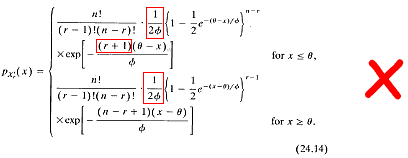Unfortunately, this solution is incorrect.   mathStatica Solution To obtain the correct solution, we first enter the pdf of our Laplace random variable into the computer: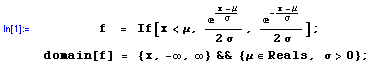Then, the pdf of the r th order statistic is given by mathStatica as: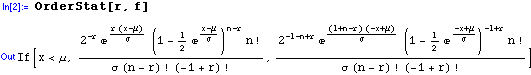It can also be shown that the subsequent equations in Johnson, Kotz and Balakrishnan (1995), namely (24.15), (24.17) and (24.18) are all incorrectly deflated by a factor of two.Pareto Distribution: Joint Order Statistics Textbook Error Let random variable X have a Pareto(a, k) distribution. Johnson, Kotz and Balakrishnan (1994, p.599) give the joint pdf of the r th and s th order statistics, for r < s, as: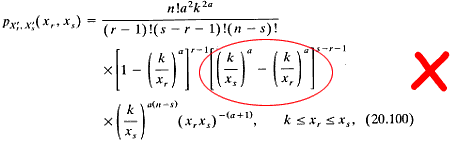This can yield negative values!   mathStatica Solution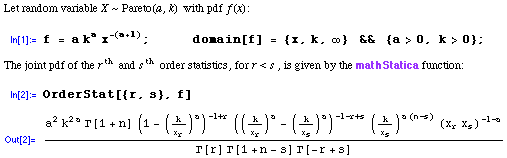Chi-squared Distribution: The Mean Deviation Textbook Error Johnson, Kotz and Balakrishnan (1994, p.420) give the mean deviation of a chi-squared random variable as:mathStatica Solution With mathStatica, it is easy to show that the circled '1' should be a '2', as shown in Rose and Smith (2002, p.422).Moments of Moments: Product cumulants Textbook Error In 1928, Fisher published the product cumulants of the k-statistics, which are now listed in reference bibles such as Stuart and Ord (1994). Here is the solution to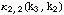as derived by Fisher in 1928 and listed in Stuart and Ord (1994, equation 12.70):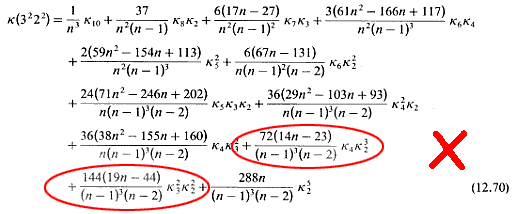Unfortunately, this known textbook 'solution' is still incorrect after more than 70 years [Rose and Smith (2002, pp. 269-271) provide a proof].  mathStatica Solution Here is the correct solution as derived by mathStatica: (the second and third terms of the output correct the old mistake)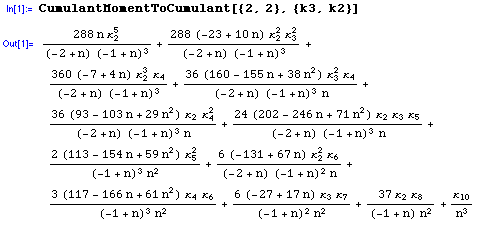There is also a small typographic error in Stuart and Ord equation 12.66: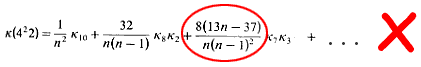which should correctly be 8(13n - 17) rather than 8(13n - 37).Bivariate Half-Normal Distribution: Regression Function Textbook Error Kotz, Balakrishnan and Johnson (2000, p.327) consider the bivariate half-Normal distribution whose marginal distributions are both half-Normal. They provide the pdf and the regression function: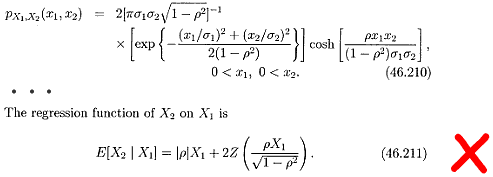where Z(.) denotes the pdf of a N(0,1) random variable. Unfortunately, this solution is wrong.  mathStatica Solution To obtain the correct solution, we start by entering the joint pdf into the computer:Then, the regression function can be derived in two steps with mathStatica: (i) Derive the conditional pdf of X2 given X1 = x1: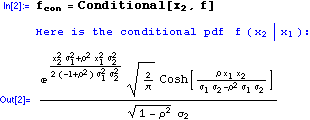with domain of support: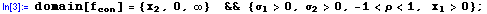(ii) Derive the regression function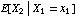: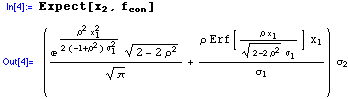Ta dah! The following diagram compares the incorrect textbook solution (KBJ 2000) with the mathStatica solution, when the variances are 1 and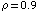: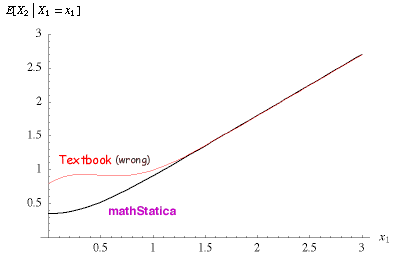Johnson Family: Third Central Moment Textbook Error Stuart and Ord (1994, Section 6.35) give the third central moment of Johnson's SU   system as: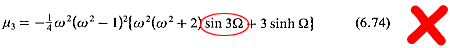mathStatica Solution Rose and Smith (2002, p.169) derive these moments using mathStatica. It appears a small typo has crept into Stuart and Ord and that the circled 'sin' function should be 'sinh'.Bivariate Exponential Distribution Textbook Error Spanos (1999, p.186) provides the following details on a Gumbel bivariate Exponential distribution: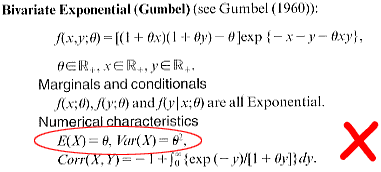Unfortunately, the solutions in Spanos for the mean and variance are wrong.  mathStatica Solution To obtain the correct solution, we start by entering the bivariate pdf into mathStatica:The correct mean and variance are then obtained as follows: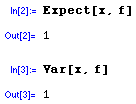As an aside, we can also do a neater job deriving the correlation between X and Y: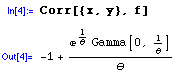Bivariate Uniform Distribution - à la Morgenstern Textbook Error Stuart and Ord (1994, p.267, Example 7.4) consider a bivariate Uniform distribution: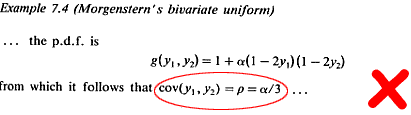mathStatica Solution To obtain the correct solution, we first enter the bivariate pdf into mathStatica: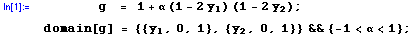Then, derive Cov(Y1 , Y2 ):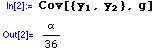The correlation between Y1 and Y2 is:Right-Censored Exponential Distribution: Mean Textbook Error Stuart and Ord (1994, p.214, Exercise 5.29) consider a right-censored Exponential distribution: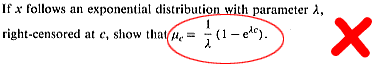mathStatica Solution We start by entering the Exponential pdf into mathStatica: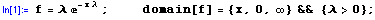Letdenote an Exponential random variable right-censored at c.   We enter this into the computer as:Then, the mean of a right-censored Exponential random variable is simply: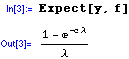which is the correct answer.Multivariate Moments of Moments Previously 'known' solution Cook (1951) published results for many product cumulants of the bivariate k-statistics. Overall, Cook's paper in Biometrika is remarkably accurate. However, there is one error in the final part of Cook's solution to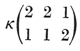, as published on pages 193 - 194 of Biometrika:The final term is in error.  mathStatica Solution Let  k21  denote the  KStatistic[{2,1}],   and  k12  denote  KStatistic[{1,2}] . Then the correct solution is easily derived by mathStatica (v2 or later) as: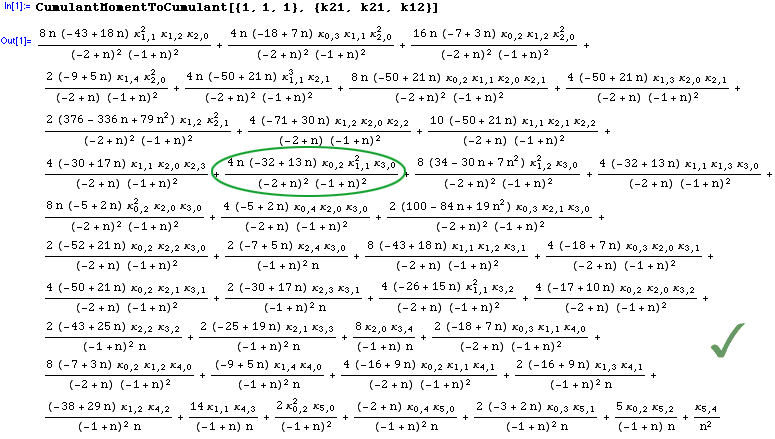Clayton copula Textbook Error Nelsen (2006, exercise 4.17) provides an algorithm that yields a Clayton copula. Unfortunately, this algorithm contains a small error (also in the first edition as exercise 4.15):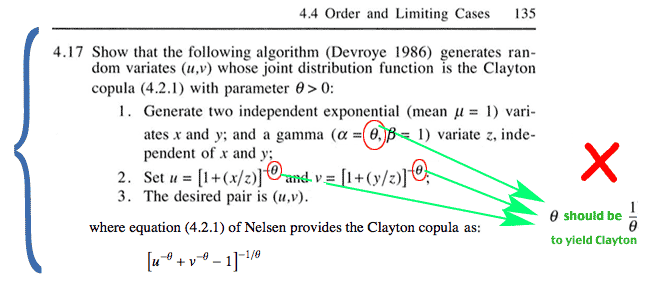mathStatica Solution We shall illustrate the error by solving the entire exercise, exactly as posed by Nelsen ... Step 1: By independence, the joint pdf of X, Y and Z is f(x, y, z):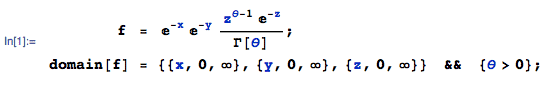Step 2: The joint pdf of (U, V, W = Z) is:with domain of support: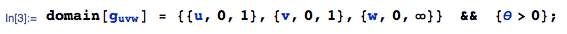Step 3: We desire the marginal joint pdf of (U, V), which is:with domain of support:That’s it. Well, almost. The first paragraph of the exercise asks us to find the joint distribution function of (U, V).i.e. find P(U < u, V < v):But ... this expression is not equal to the Clayton copula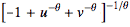. The green text above shows how to correct Nelsen’s exercise so that the method will work correctly.Shifted Exponential Textbook Error   —   found by Prof Bruce McCullough Devore and Berk (2012, Exercise Section 4.2: 19) consider a shifted Exponential model for traffic: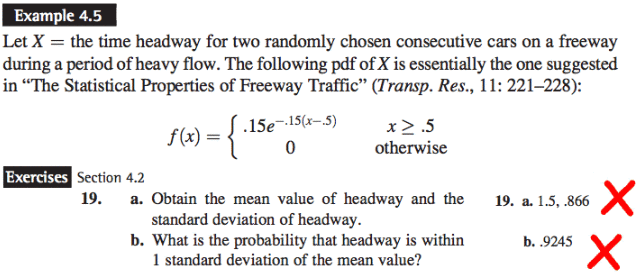mathStatica Solution Step 1: The pdf of X, say f(x), is:Part (a): The exact mean and standard deviation are: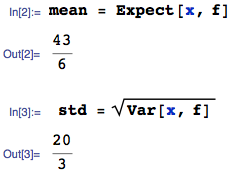... which are approximately 7.16 (not .15) and 6.66 (not .866) respectively. Part (b): The probability that X is within one standard deviation of the mean is:... which is approximately 0.864665 (not .9245).Reference List Cook, M. B. (1951)     Bi-variate k-statistics and cumulants of their joint sampling distribution     Biometrika, volume 38, 179-195 Devore, J. L. and Berk, K. N. (2012)     Modern Mathematical Statistics with Applications, 2nd edition, Springer Fisher, R. A. (1928)     Moments and product moments of sampling distributions     Proceedings of the London Mathematical Society - series 2, volume 30, 199-238 Johnson, Kotz, Balakrishnan (1994)     Continuous Univariate Distributions, volume 1, 2nd edition Johnson, Kotz, Balakrishnan (1995)     Continuous Univariate Distributions, volume 2, 2nd edition Kotz, Balakrishnan, Johnson (2000)     Continuous Multivariate Distributions, volume 1, 2nd edition Nelsen, R. B. (2006)     An Introduction to Copulas, 2nd edition (also 1st edition) Ord, J. K. (1972)     Families of Frequency Distributions Spanos, A. (1999)     Probability Theory and Statistical Inference: Econometric Modeling with Observational Data Stuart, A. and Ord, J. K. (1994)     Kendall's Advanced Theory of Statistics - volume 1, 6th edition